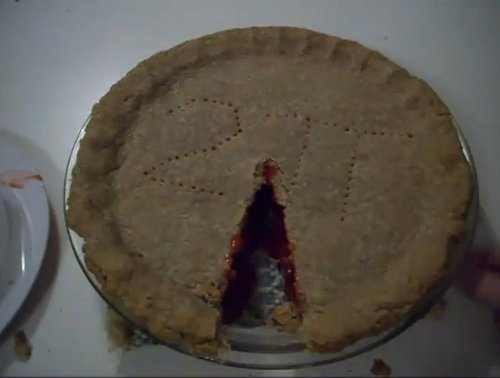# Perfect Day for a Pi FightIf you’re a fan of mathematical quirks, today is a doubly momentous date.

First of all, 6/28 is the only day of the year where both the day of the month and the number of the month are perfect numbers: that is, a number that equals the total of the numbers it can be divided by, such as 1+2+3=6 or 1+2+4+7+14=28.

But it’s also been adopted as Tau Day, the counterpart to the idea of March 14 (3/14, as in 3.14) being Pi Day. The idea comes from “theoretical physicist” Michael Hartl who has a particular bugbear: he hates the idea of putting the emphasis on the ratio of circumference to diameter.

According to Hartl, this is the wrong way to approach matters as the essence of a circle is the various points around its circumference, each of which is the same distance from the centre, namely the radius. He suggests that using pi causes an unnecessary additional step with pi having to be doubled for many calculations.

In fact, so adamant is Hartl about his idea, he argues that the “failings” of pi are the main reason we traditionally measure angles in degrees rather than radians (the angle at which the relevant arc, or section of the circle edge, is equal to the radius.)

In response, Hartl proposes making our main circle-related ratio be 6.28 (and many digits thereafter, the number being signified by Greek letter Tau), which represents the number of radians in a turn (a 360 degree rotation), which is of course also the circumference:radius ratio and 2 x pi.

And even if that all sounds a little pointless/pompous/pedantic (delete as appropriate) for your tastes, you still might be tempted by a special Tau Day party to be held at the Cahill Center for Astronomy and Astrophysics in Pasadena tonight. Entry is free.

But you are asked to bring two pies.

(Image credit: Pi Is Still Wrong)

-Geeky T-Shirt Sale: 1000s of TEES at Just \$16 Each!